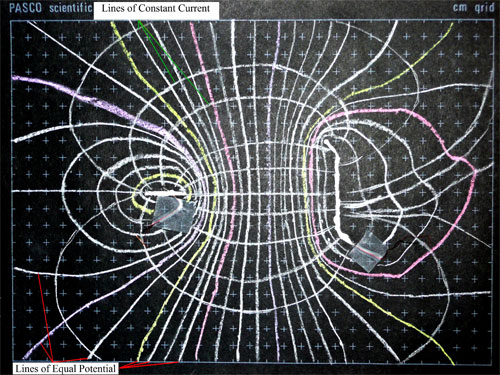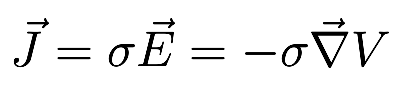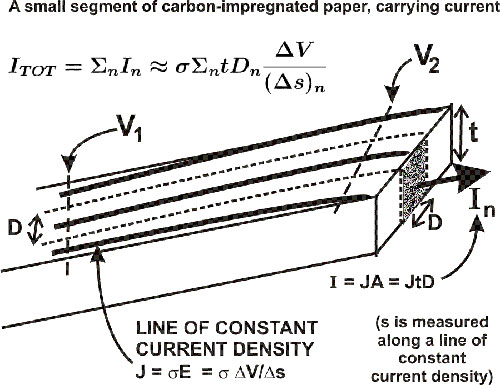# Experiment of The Month

## Current Density in a Conducting Sheet

Carbon impregnated paper is used in an exercise to map equipotential "surfaces" as lines on the two-dimensional sheet. Two electrodes are painted on the paper with silver-loaded glue, a potential is applied across the electrodes, and a voltmeter is used to map lines of constant potential. We do this exercise in intermediate laboratory as a way of introducing the ideas of surface studies, for later application in our senior projects on sculptured thin films.

The photo below shows one of the resulting maps. The electrodes are a horizontal bar on the left and a "backwards S" on the right.Equipotential lines are marked with colored chalk. They run almost vertically in the center of the figure, and wrap around the electrodes without touching at the left and right sides of the figure. Lines of constant current density are sketched perpendicular to the lines of constant potential, following the rule:where J is current density, sigma is conductivity, E is electric field, and V is electric potential. The current direction is the same as (-) the gradient of the potential.

To estimate the conductivity of the paper from this map, we measured (in addition to potential) the total current through the electrodes and the thickness of the paper. We assumed that the conductivity is uniform throughout the paper. We focused on the region where most of the current flows, near the central distance between electrodes. We assumed that the current density is uniform in the region surrounding one of our lines of constant current density.

We estimated the current associated with a particular line of constant current density using the figure below:V1 and V2 are lines of constant potential. D is measured between the half-way points separating adjacent lines of constant current density. In this way, each constant current density line is associated with an element of current. The sum of these current elements must be equal to the total current through the electrodes, which we measured.

For a given pair of constant potential lines, D and the distance Ds between those lines are typically different for each different line of constant current density. (We used the average value of D.)

This model for the current distribution was confirmed by measuring the conductivity using a long narrow strip of the same conducting paper.

## Experiment Of The Month

View past labs experiments.